# Texas Go Math Grade 4 Lesson 13.6 Answer Key Find and Draw Lines of Symmetry

Refer to our Texas Go Math Grade 4 Answer Key Pdf to score good marks in the exams. Test yourself by practicing the problems from Texas Go Math Grade 4 Lesson 13.6 Answer Key Find and Draw Lines of Symmetry.

## Texas Go Math Grade 4 Lesson 13.6 Answer Key Find and Draw Lines of Symmetry

Essential Question

How do you find lines of symmetry?
We can find the lines of symmetry if a shape has a Line of Symmetry by folding it. When the folded part settles perfectly on top (all edges should match), then the folding line is a Line of Symmetry.

Activity 1 Find lines of symmetry.

Materials

• isometric and square dot paper
• straightedge

STEP 1 Draw a triangle like the one shown, so all sides have equal length.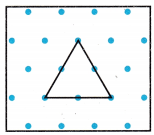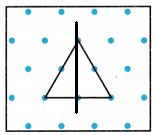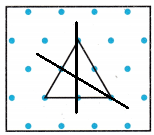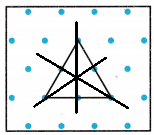STEP 2 Fold the triangle in different ways to test for line symmetry. Draw along the fold lines that are lines of symmetry.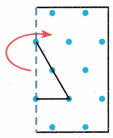Is there a line of symmetry if you fold the paper horizontally?
No.

Explanation: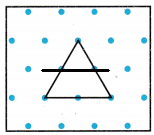If we fold the paper horizontally there will be no line of symmetry.

STEP 3
Repeat the steps for each polygon shown. Complete the table.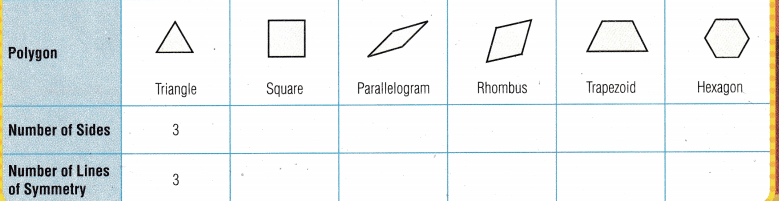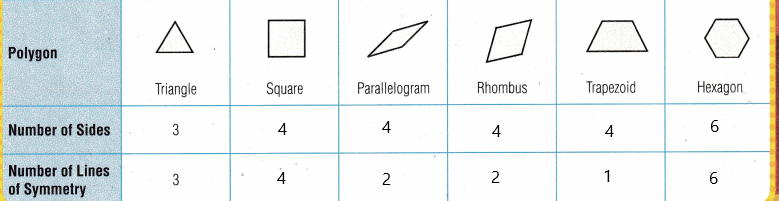In a regular polygon, all sides are of equal length and all angles are equal. What do you notice about the number of lines of symmetry in regular polygons?
A regular pentagon has five sides and five lines of symmetry. The number of lines of symmetry in a regular polygon is equal to the number of sides.

Math Talk

Mathematical Processes
How many lines of symmetry does a circle have? Explain.
There are an infinite number of lines through the center, therefore the circle has an infinite number of lines of symmetry.

Explanation:
When the circle is folded over a line of symmetry, the parts of the circle on each side of the line match up. This means any line that passes through the center of the circle will be a line of symmetry.

ERROR Alert: To avoid errors, you may use a mirror to check for line symmetry.

Activity 2 Make designs that have line symmetry.

Materials

• pattern blocks

Make a design by using more than one pattern block. Record your design. Draw the line or lines of symmetry.

Make a design with 2 lines of symmetry.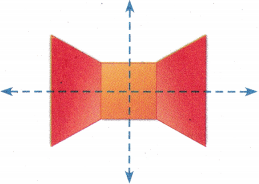Make a design with 1 line of symmetry.

Make a design with more than 2 lines of symmetry.

Make a design with zero lines of symmetry.

Share and Show

Question 1.
The figure at the right has line symmetry. Draw the 2 lines of symmetry.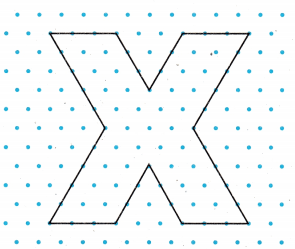Tell whether the figure appears to have zero lines, 1 line, or more than 1 line of symmetry. Write zero, 1, or more than 1.

Question 2.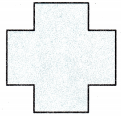More than 1 line of symmetry.

Explanation:
The given figure has more than one line of symmetry.

Question 3.More than 1 line of symmetry.

Explanation:
The given figure has more than one line of symmetry.

Question 4.one line of symmetry

Explanation:
The given figure has one line of symmetry.

Question 5.Zero line of symmetry

Explanation:
The given figure has zero line of symmetry.

Math Talk

Mathematical Processes
Explain how you can lines of symmetry for a figure.

Problem Solving

Use the chart for 6-8.Question 6.
Which letters appear to have only 1 line of symmetry?

Question 7.
Which letters appear to have zero lines of symmetry?

Question 8.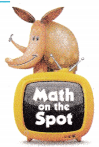H.O.T. The letter C has horizontal symmetry. The letter A has vertical symmetry. Which letters appear to have both horizontal and vertical symmetry?

Question 9.
H.O.T. Justify Sense or Nonsense? Jeff says that the figure has only 2 lines of symmetry.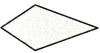Does his statement make sense? Explain.
Yes, it makes sense.

Explanation:
The given figure has only 2 lines of symmetry and it makes sense.

Question 10.
H.O.T. Communicate Multi-Step Draw a figure that has at least 2 lines of symmetry. Then write instructions that explain how to find the lines of symmetry.

Question 11.
How many lines of symmetry does the shell have?(A) 0
(B) 3
(C) 2
(D) 1
1

Explanation:
The figure has one line of symmetry.

Question 12.
Which shows all the lines of symmetry for the figure below?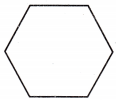(A)(B)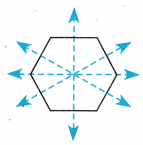(C)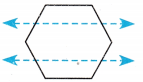(D)Option D has shows all the lines of symmetry for the figure.

Question 13.
Which describes the figure?(A) zero lines of symmetry
(B) 2 lines of symmetry
(C) more than 2 lines of symmetry
(D) 1 line of symmetry
More than 2 lines of symmetry

Explanation:
The figure describes it has more than 2 lines of symmetry.

TEXAS Test Prep

Question 14.
How many lines of symmetry does the figure to the right have?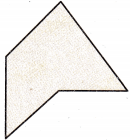(A) 10
(B) 3
(C) 5
(D) 0
3

Explanation:
The given figure has three lines of symmetry.

### Texas Go Math Grade 4 Lesson 13.6 Homework and Practice Answer Key

Tell whether the shape appears to have zero lines, I line, or more than 1 line of symmetry. Write zero, 1, or more than 1.

Question 1.More than 1 line of symmetry

Explanation:
The given shape has more than 1 line of symmetry.

Question 2.Zero line of symmetry.

Explanation:
The given figure has zero line of symmetry.

Question 3.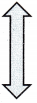One line of symmetry.

Explanation:
The given figure has one line of symmetry.

Question 4.More than 1 line of symmetry.

Explanation:
The given shape has more than 1 line of symmetry.

Problem Solving

Use the poster for 5-6.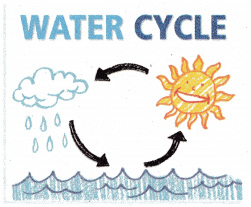Question 5.
Erica is cutting out letters for a science poster. Which letters on Erica’s poster appear to have only 1 line of symmetry?
W, A, E, C, Y, C, E

Explanation:
The letters on Erica’s poster appear to have only 1 line of symmetry are W, A, E, C, Y, C, E

Question 6.
Which letters on Erica’s poster appear to have zero lines of symmetry?
L

Explanation:
The letter L on Erica’s poster appears to have zero lines of symmetry.

Question 7.
Write your own poster words. Include letters that have zero, one, and two lines of symmetry. Then draw the lines of symmetry.

Lesson Check

Question 8.
How many lines of symmetry does the jar have?(A) 0
(B) 1
(C) 2
(D) 3
2

Explanation:
The jar has 2 lines of symmetry.

Question 9.
Which describes this figure?(A) zero lines of symmetry
(B) 1 line of symmetry
(C) 2 lines of symmetry
(D) more than 2 lines of symmetry
1 line of symmetry

Explanation:
The given figure has 1 line of symmetry.

Question 10.
Which shows all the lines of symmetry for the figure?(A)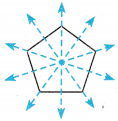(B)(C)(D)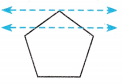Option A

Explanation:
Option A shows all the lines of symmetry for the figure.

Question 11.
How many lines of symmetry does the figure have?(A) 3
(B) 2
(C) 1
(D) 0
Option D

Explanation:
The given figure has zero lines of symmetry.

Question 12.
Multi-Step Which statement about the number of lines of symmetry is true?(A) They are greater than the number of sides.
(B) They are less than the number of angles.
(C) They are the same as the number of sides.
(D) They are greater than the number of angles.

Question 13.
Multi-Step Lance drew this figure with two lines of symmetry. Which statement is true about the number of lines of symmetry?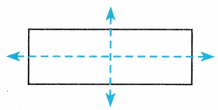(A) They are the same as the number of sides.
(B) They are greater than the number of sides.
(C) They are less than the number of pairs of sides of equal length.
(D) They are the same as the number of pairs of sides of equal length.